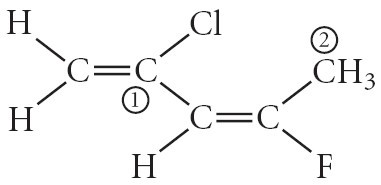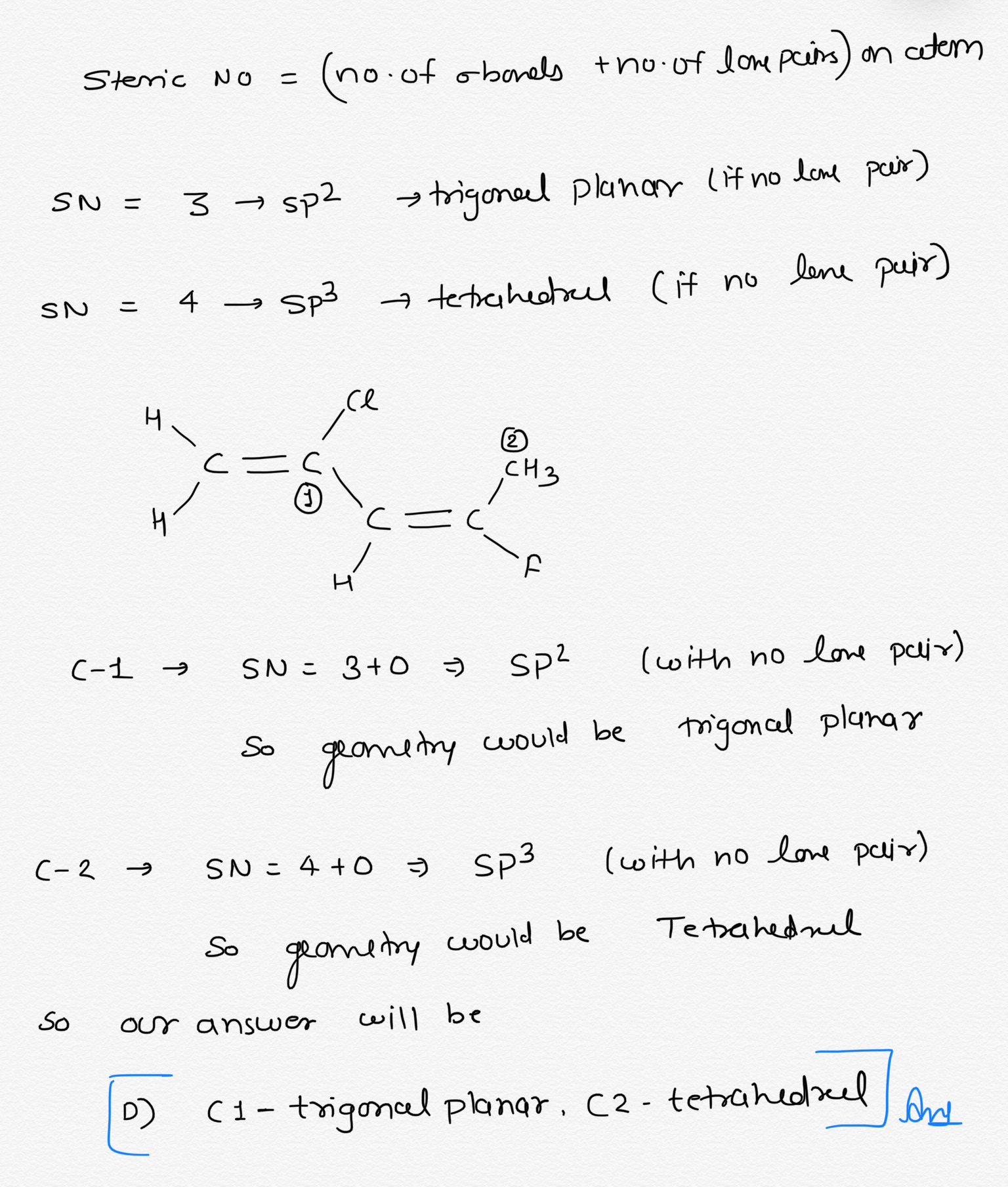# Consider the molecule below. determine the molecular geometry at each of the 2 labeled carbons.

Consider the molecule below. Determine the molecular
geometry at each of the 2 labeled carbons. molecular
geometryA) C1 = tetrahedral, C2 = linear B) C1 = trigonal planar, C2 = bent C) C1 = bent, C2 = trigonal planar D) C1 = trigonal planar, C2 = tetrahedral E) None of the above CEC

Sterie No – (no of oborels +no. of lone pcins) on atem SN= 3 sp2 trigoneel planar (if no lone pair) lene SN= 4 tetrahedrul (if no puir) Sp3 CH3 – (-1 SN= 3+0 → sp2 So geometry would be (with no lone pair) trigoncal planas (-2 SN=4+0 sp (with no lone pair) So geometry would be Tetrahedral our answer will be 1 C1- trigoncel planar. C2 – tetrahedzeel love so